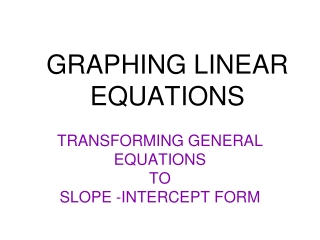DownloadDownload PresentationGRAPHING LINEAR EQUATIONS

# GRAPHING LINEAR EQUATIONS

Télécharger la présentation## GRAPHING LINEAR EQUATIONS

- - - - - - - - - - - - - - - - - - - - - - - - - - - E N D - - - - - - - - - - - - - - - - - - - - - - - - - - -
##### Presentation Transcript

1. GRAPHING LINEAR EQUATIONS • TRANSFORMING GENERAL EQUATIONS • TO • SLOPE -INTERCEPT FORM

2. Example: Y-intercept Y= ⅔x -4 Graphing a linear equation in slope-intercept form:y=mx+b ⅔x -4 run: 3 units ● RISE: 2 units ●

3. Y-intercept YOUR TURN!slope-intercept form:y=mx+b ● -⅗x Y= -⅗x +2 +2 down: 3 units ● run: 5 units

4. -2x 3 3 -2x 3 Y-intercept Graphing a linear equation in Standard form:Ax + By = C ● 2x+3y= 12 down: 2 units ● run: 3 units 3y= -2x +12 -⅔x y= -⅔x +4 +4

5. -15x -3 -3 -15x -3 Y-intercept Your Turn!Standard form:Ax + By = C 15x-3y= 9 run: 1 unit ● Rise: 5units -3y= -15x +9 ● y= 5x -3 5x -3

6. Classwork: A. Convert the following standard equation of a line intoslope-intercept form